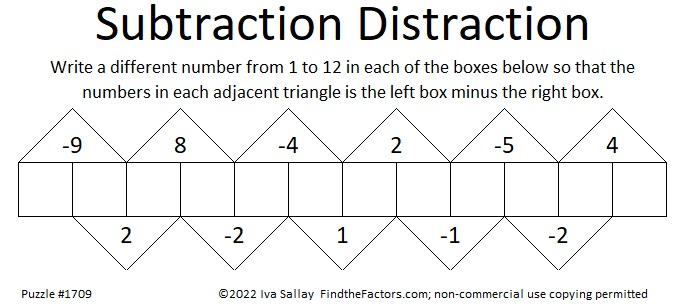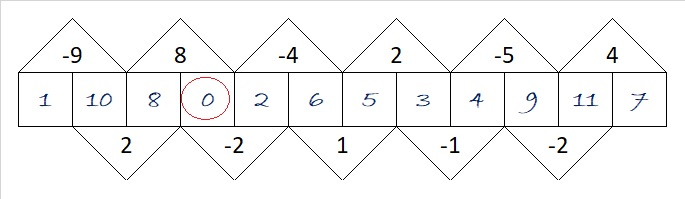# 1709 Sometimes “Guess and Check” Is a Good Strategy

Contents

### Today’s Puzzle:

Most of the puzzles I publish are logic puzzles, and I encourage you to find the logic of the puzzle and not guess and check.  However, guess and check is a legitimate strategy in mathematics, and it is a legitimate strategy to solve this particular puzzle.Since one of the clues is -9, we know that the two boxes under it must be [1, 10], [2, 11], or [3, 12].

Suppose you assume it’s 1 – 10 = -9. If you fill out the rest of the boxes you would get:You know that isn’t right because zero is not a number from 1 to 12. No problem. Simply add one to each of the numbers you wrote in, and the puzzle will be solved with only numbers from 1 to 12.

Suppose you assumed it’s 3 -12 = -9. The rest of the boxes would look like this:Again, 13 is not included in the numbers from 1 to 12, but you can fix it by subtracting 1 from each of the numbers you wrote in. Easy Peasy.

### Factors of 1709:

• 1709 is a prime number.
• Prime factorization: 1709 is prime.
• 1709 has no exponents greater than 1 in its prime factorization, so √1709 cannot be simplified.
• The exponent in the prime factorization is 1. Adding one to that exponent we get (1 + 1) = 2. Therefore 1709 has exactly 2 factors.
• The factors of 1709 are outlined with their factor pair partners in the graphic below.How do we know that 1709 is a prime number? If 1709 were not a prime number, then it would be divisible by at least one prime number less than or equal to √1709. Since 1709 cannot be divided evenly by 2, 3, 5, 7, 11, 13, 17, 19, 23, 29, 31, 37, or 41, we know that 1709 is a prime number.

### More About the Number 1709:

1709 is the sum of two squares:
35² + 22² = 1709.

1709 is the hypotenuse of a Pythagorean triple:
741-1540-1709, calculated from 35² – 22², 2(35)(22), 35² + 22².

Here’s another way we know that 1709 is a prime number: Since its last two digits divided by 4 leave a remainder of 1, and 35² + 22² = 1709 with 35 and 22 having no common prime factors, 1709 will be prime unless it is divisible by a prime number Pythagorean triple hypotenuse less than or equal to √1709. Since 1709 is not divisible by 5, 13, 17, 29, 37, or 41, we know that 1709 is a prime number.

This site uses Akismet to reduce spam. Learn how your comment data is processed.